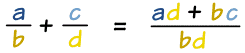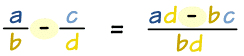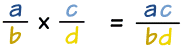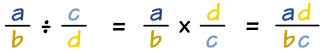# 代数里的分数

## 分数加法（去公分母看看为什么）。

### 例子：

x 2 + y 5 = (x)(5) + (2)(y) (2)(5)

= 5x+2y 10

### 例子：

x + 4 3 + x − 3 4 = (x+4)(4) + (3)(x−3) (3)(4)

= 4x+16 + 3x−9 12

= 7x+7 12

## 分数减法### 例子：

x + 2 x  −  x x − 2  =  (x+2)(x−2) − (x)(x) x(x−2)

(x2 − 22) − x2 x2 − 2x

−4 x2 − 2x

## 分数乘法### 例子：

3x x−2  ×  x 3  =  (3x)(x) 3(x−2)

3x2 3(x−2)

x2 x−2

## 分数除法### 例子：

3y2 x+1  ÷  y 2  =  3y2 x+1  ×  2 y

= (3y2)(2) (x+1)(y)

= 6y2 (x+1)(y)

6y x+1